Tamilnadu State Board New Syllabus Samacheer Kalvi 12th Maths Guide Pdf Chapter 4 Inverse Trigonometric Functions Ex 4.5 Textbook Questions and Answers, Notes.

## Tamilnadu Samacheer Kalvi 12th Maths Solutions Chapter 4 Inverse Trigonometric Functions Ex 4.5

Question 1.
Find the value, if it exists. If not, give the reason for non-existence.
(i) sin-1 (cos π)
(ii) tan-1(sin($$\frac {-5π}{2}$$))
(iii) sin-1 [sin 5]
Solution:
(i) sin-1 (cos π) = sin-1 (-1) = -sin-1 (1) = –$$\frac {π}{2}$$ [∵ cos π = -1]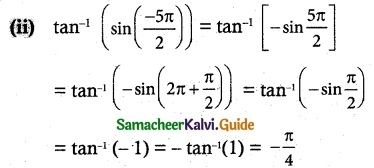(iii) sin-1 [sin 5]
–$$\frac {π}{2}$$ ≤ sin-1 5 ≤ $$\frac {π}{2}$$
-3$$\frac {π}{2}$$ ≤ 5 ≤ 2π
–$$\frac {π}{2}$$ ≤ 5 – 2π ≤ 0 ≤ $$\frac {π}{2}$$
sin(5 – 2π) = sin 5
sin-1 (sin 5) = 5 – 2πQuestion 2.
Find the value of the expression in terms of x, with the help of a reference triangle.
(i) sin (cos-1(1 – x))
(ii) cos (tan-1 (3x – 1))
(iii) tan (sin-1(x + $$\frac {π}{2}$$))
Solution:
(i) sin (cos-1(1 – x)) = sin [cos-1(adj/hyp)]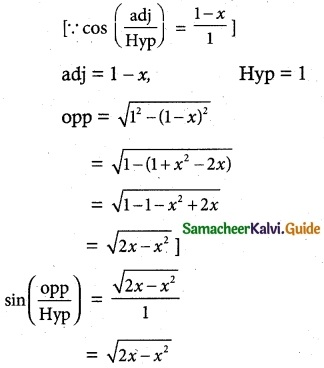(ii) cos (tan-1(3x – 1)) = cos [opp/adj]
Let θ = tan-1(3x – 1)
tan θ = 3x- 1
1 + tan² θ = 1 +(3x – 1)²
sec² θ = 9x² – 6x + 2
sec θ = $$\sqrt{9x² – 6x + 2}$$
cos θ = $$\frac{1}{\sqrt{9x² – 6x + 2}}$$
⇒ cos (tan-1(3x – 1)) = $$\frac{1}{\sqrt{9x² – 6x + 2}}$$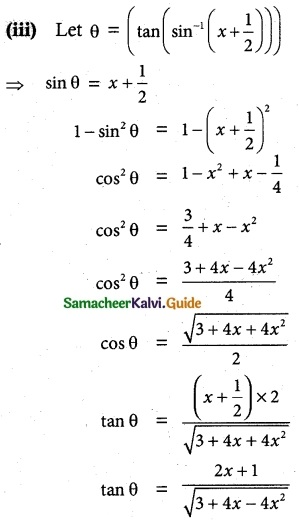Question 3.
Find the value of
(i) sin-1(cos(sin-1($$\frac {√3}{2}$$)))
(ii) cot(sin-1 $$\frac {3}{5}$$ + sin-1$$\frac {4}{5}$$)
(iii) tan(sin-1 $$\frac {3}{5}$$ + cot-1$$\frac {3}{2}$$)
Solution: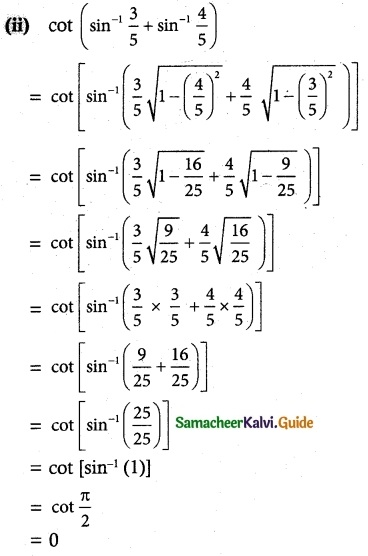Question 4.
(i) tan-1$$\frac {2}{11}$$ + tan-1 $$\frac {7}{24}$$ = tan-1 $$\frac {1}{2}$$
(i) tan-1$$\frac {3}{5}$$ + cos-1 $$\frac {12}{13}$$ = sin-1 $$\frac {16}{65}$$
Solution: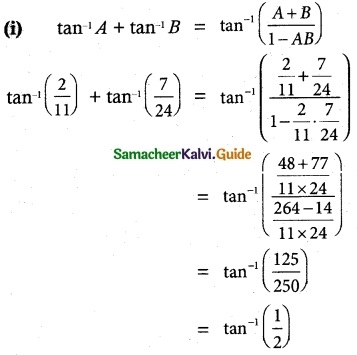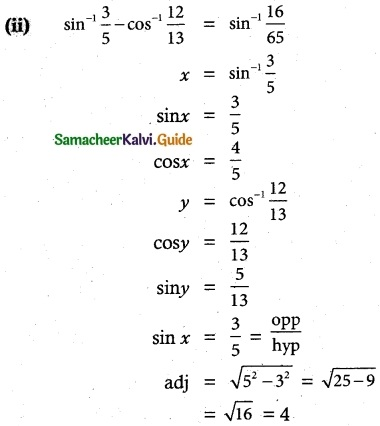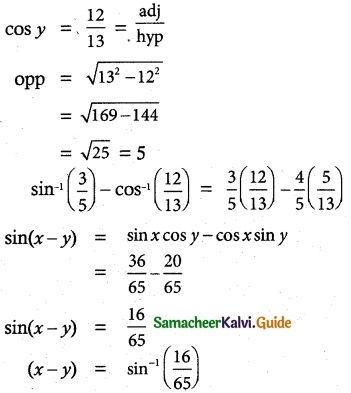Question 5.
Prove that
tan-1 x + tan-1 y + tan-1 z = tan-1 ($$\frac {x+y+z-xyz}{1-xy-yz-zx}$$)
Solution:
tan-1 x + tan-1 y + tan-1 z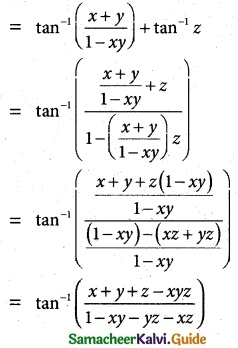Question 6.
tan-1 x + tan-1 y + tan-1 z = π, show that x + y + z = xyz
Solution:
tan-1 x + tan-1 y + tan-1 z = π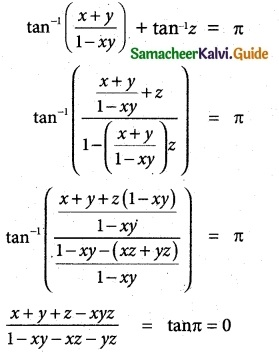x + y + z – xyz = 0
x + y + z = xyz

Question 7.
Prove that
tan-1 x + tan-1 $$\frac {2x}{1-x^2}$$ = tan-1 $$\frac {3x-x^3}{1-3x^2}$$, |x| < $$\frac {1}{√3}$$
Solution: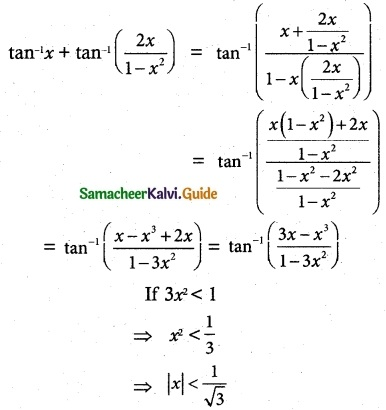Question 8.
Simplify
tan-1 $$\frac {x}{y}$$ – tan-1 $$\frac {x-y}{x+y}$$
Solution: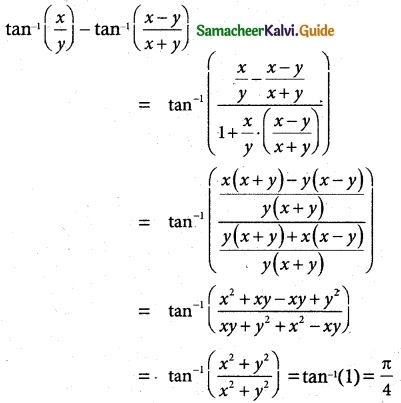Question 9.
(i) sin-1 $$\frac {5}{x}$$ + sin-1 $$\frac {12}{x}$$ = $$\frac {π}{2}$$
(ii) 2 tan-1 x = cos-1 $$\frac {1-a^2}{1+a^2}$$ – cos-1 $$\frac {1-b^2}{1+b^2}$$, a > 0, b > 0
(iii) 2 tan-1 (cos x) = tan-1 (2 cosec x)
(iv) cot-1 x – cot-1 (x + 2) = $$\frac {π}{12}$$, x > 0
Solution: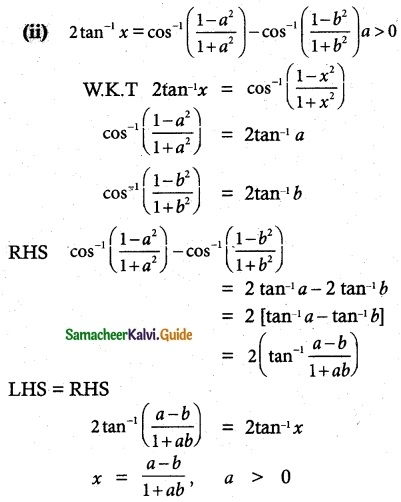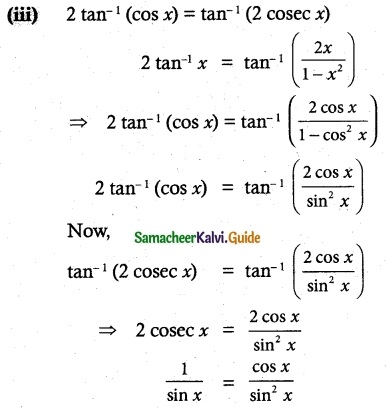sin² x = sin x cos x
⇒ sin x cos x – sin² x = 0
⇒ sin x(cos x – sin x) = 0
sin x = 0 (or) cos x – sin x = 0
⇒ x = nπ, n ∈ Z, (or) cos x = sin x
tan x = 1 = tan $$\frac {π}{4}$$
⇒ x = nπ + $$\frac {π}{4}$$, n ∈ Z⇒ (x + 1)² = 4 + 2√3
⇒ (x + 1)² = 1 + 3 + 2√3
⇒ (x + 1)² = (1 + √3)²
⇒ x + 1 = 1 + √3
∴ x = √3Question 10.
Find the number of the solutions of the equations
tan-1(x – 1) + tan-1 x + tan-1(x + 1) = tan-13x
Solution:
tan-1(x – 1) + tan-1 x + tan-1(x + 1)
= tan-1(x – 1) + tan-1(x + 1) + tan-1x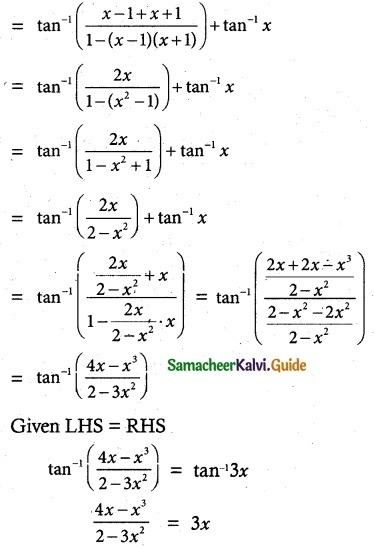4x – x³ = 6x – 9x³
8x³ = 2x
8x³ – 2x = 0
2x(x² – 1) = 0
x = 0, x² = 1
x = ±1
Number of solutions are three (0, 1 -1)